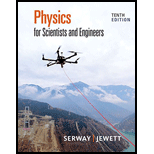Chapter 25, Problem 19P### Physics for Scientists and Enginee...

10th Edition
Raymond A. Serway + 1 other
ISBN: 9781337553278

#### Solutions

Chapter
Section### Physics for Scientists and Enginee...

10th Edition
Raymond A. Serway + 1 other
ISBN: 9781337553278
Textbook Problem

# Two identical parallel-plate capacitors, each with capacitance 10.0 μF, are charged to potential difference 50.0 V and then disconnected from the battery. They are then connected to each other in parallel with plates of like sign connected. Finally, the plate separation in one of the capacitors is doubled. (a) Find the total energy of the system of two capacitors before the plate separation is doubled. (b) Find the potential difference across each capacitor after the plate separation is doubled. (c) Find the total energy of the system after the plate separation is doubled. (d) Reconcile the difference in the answers to parts (a) and (c) with the law of conservation of energy.

(a)

To determine
The total energy of the system of two capacitors before the plate separation is doubled.

Explanation

Given info: The capacitance of each capacitor is 10.0μF , the potential difference is 50.0V .

Write the expression to calculate the total energy of the system of two capacitors.

UE=U1+U2 (1)

Here,

U1,U2 is the energy of single capacitor.

The energy of capacitor is,

U=12C(ΔV)2

Here,

C is the capacitance of each capacitor.

ΔV is the potential deference.

Substitute 12C(ΔV)2 for U1,U2 in equation (1).

UE=12C(ΔV)2+12C(ΔV)2=C(ΔV)2

Substitute 10

(b)

To determine
The potential difference across each capacitor after the plate is separation is doubled.

(c)

To determine
The total energy of the system after the plate is separation is doubled.

(d)

To determine
The result by comparing the answer in part (a) and (c).

### Still sussing out bartleby?

Check out a sample textbook solution.

See a sample solution

#### The Solution to Your Study Problems

Bartleby provides explanations to thousands of textbook problems written by our experts, many with advanced degrees!

Get Started

## Additional Science Solutions

#### Find more solutions based on key concepts